## Improved Contrastive Divergence Training of Energy Based Models

### ICML 2021

1 MIT CSAIL    2 Google Brain

We propose several different techniques to improve contrastive divergence training of energy-based models (EBMs). We first show that a gradient term neglected in the popular contrastive divergence formulation is both tractable to estimate and is important to avoid training instabilities in previous models. We further highlight how data augmentation, multi-scale processing, and reservoir sampling can be used to improve model robustness and generation quality. Thirdly, we empirically evaluate stability of model architectures and show improved performance on a host of benchmarks and use cases, such as image generation, OOD detection, and compositional generation.

## Improved Training of Energy-Based Models

Energy-Based Models (EBMs) represent the likelihood of a probability distribution of data by assigning an unnormalized probability scalar (or "energy") to each input data point. This provides significant model flexibility; any arbitrary model that outputs a real number can be used as an energy model. A difficulty however, is that training EBMs is hard, as to properly maximize the likelihood of an energy function, samples must be drawn from the energy model. In this work we present a set of improvements to contrastive divergence training of EBMs, enabling more stable, high resolution generation with EBMs. In particular we propose to:

• Add a KL loss term into contrastive divergence, which corresponds to a typically ignored gradient. We show that this significantly stabilizes and improves generative performance.
• Integrate data-augmentation transitions while training EBMs to encourage mode mixing between model samples.
• Factorize generation to a set of multi-scale energy functions operating on the input.

## Contrastive Divergence

A common objective used to train EBMs is contrastive divergence. Contrastive divergence consists of the following objective: $\text{KL}(p_D(x) \ || \ p_{\theta} (x)) - \text{KL}(q_{\theta}(x) \ || \ p_{\theta}(x)).$ This objective minimizes the difference between the KL divergence of the data distribution and EBM distribution, and the KL divergence of finite number of MCMC steps on data distribution and EBM distribution. This objective has a key gradient (highlighted in red) that is often ignored. $- \left ( \mathbb{E}_{p_D(x)} \left [\frac{\partial E_{\theta}(x)}{\partial \theta} \right ] - \mathbb{E}_{q_{\theta}(x')} [\frac{\partial E_{\theta}(x')}{\partial \theta}] + {\color{red} \frac{\partial q(x')}{\partial \theta} \frac{\partial \text{KL}(q_{\theta}(x') \ || \ p_{\theta}(x'))}{\partial q_{\theta}(x')} } \right )$ We present a KL loss to capture this gradient (see our paper for details). We find that this missing gradient contributes substantially to the overall training gradient of a EBM. The addition of our proposed KL loss significantly stablizes training of EBMs and enables us to add additional architectural blocks to training.

## Data Augmentation

A difficulty when training EBMs is that underlying MCMC chains fail to mix and cover the EBM distribution. To enable more effective mixing of MCMC chains, we intersperse Langevin sampling with data augmentation transitions. This enables image sampling chains from our model to travel across large number of modes in the energy landscape. We illustrate the underlying sampling process below:## Zero Shot Compositional Generation

EBMs are able to independently compose with other EBMs, allowing us flexibly compose different models. We show that our approach enables higher resolution compositional generation across different domains. We independently train EBMs for CelebA factors of age, gender, smiling, and wavy hair. We show below that by adding each energy model in generation, we are able to gradually able to construct and change generations to exhibit each desired factor, as encoded by an individual energy function.

We can further independently train EBMs on rendering attributes of shape, size, position and rotation. By adding independent energy model in generation, we are also able to gradually construct generations that exhibit each desired factor.

## Out of Distribution Robustness

Another interesting property of EBMs is their ability to identify out-of-distribution samples not in the training data distribution by utilizing the output predicted energy. We find that our approach significantly outperforms other EBMs on the task of identifying out-of-distribution data samples.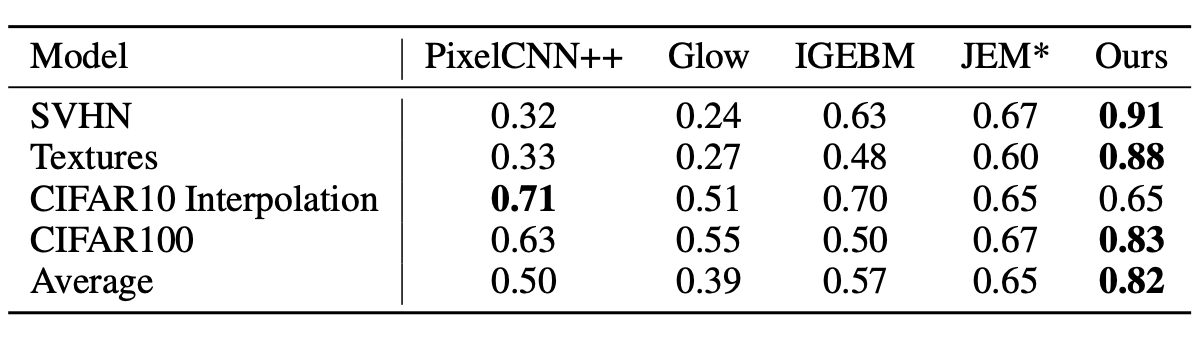## Related Projects

Check out our related projects on utilizing energy based models!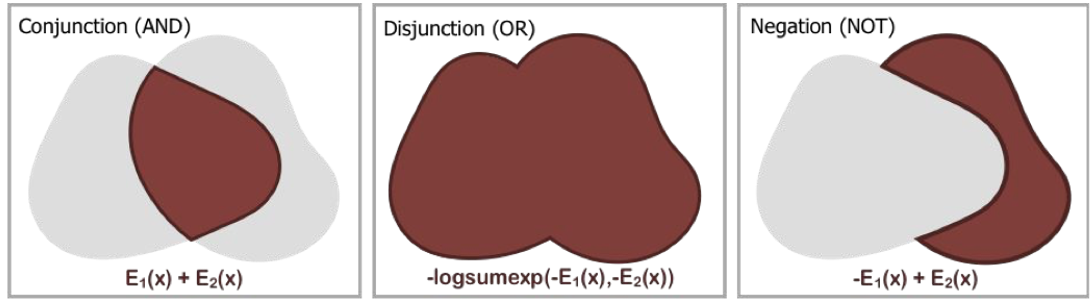We show how EBMs enable zero-shot compositional visual generation, enabling us to compose visual concepts (through operators of conjunction, disjunction, or negation) together in a zero-shot manner. Our approach enables us to generate faces given a description ((Smiling AND Female) OR (NOT Smiling AND Male)) or to combine several different objects together.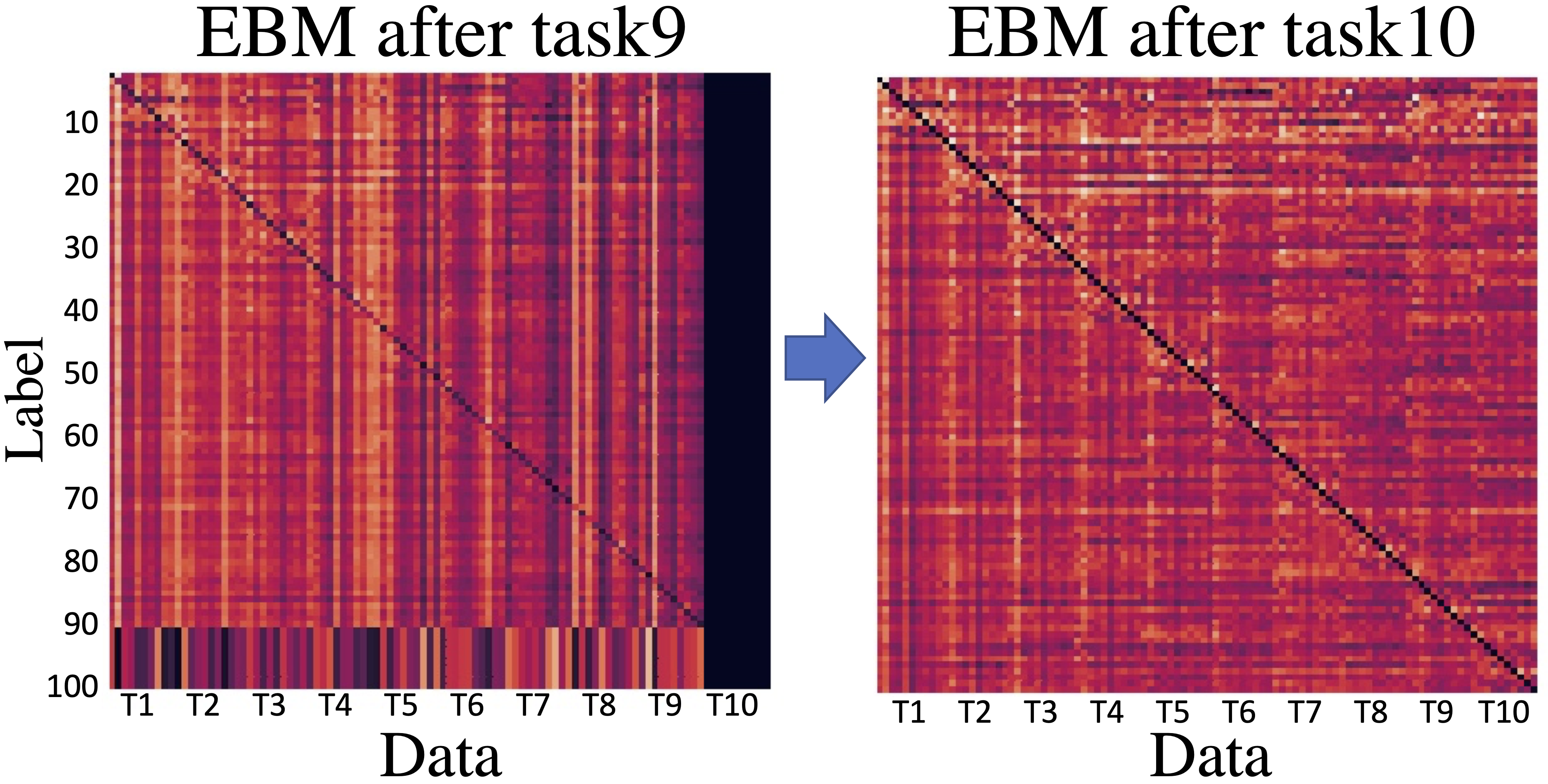We show how EBMs help provide an orthogonal approach towards tackling continual learning problems by changing the underlying training objective to causes less interference with previously learned information. Our proposed EBM formulation is simple, efficient, and outperforms baseline methods by a large margin on several benchmarks. We further show that EBMs are adaptable to a harder continual learning setting where the data distribution changes without explicitly delineated task boundaries.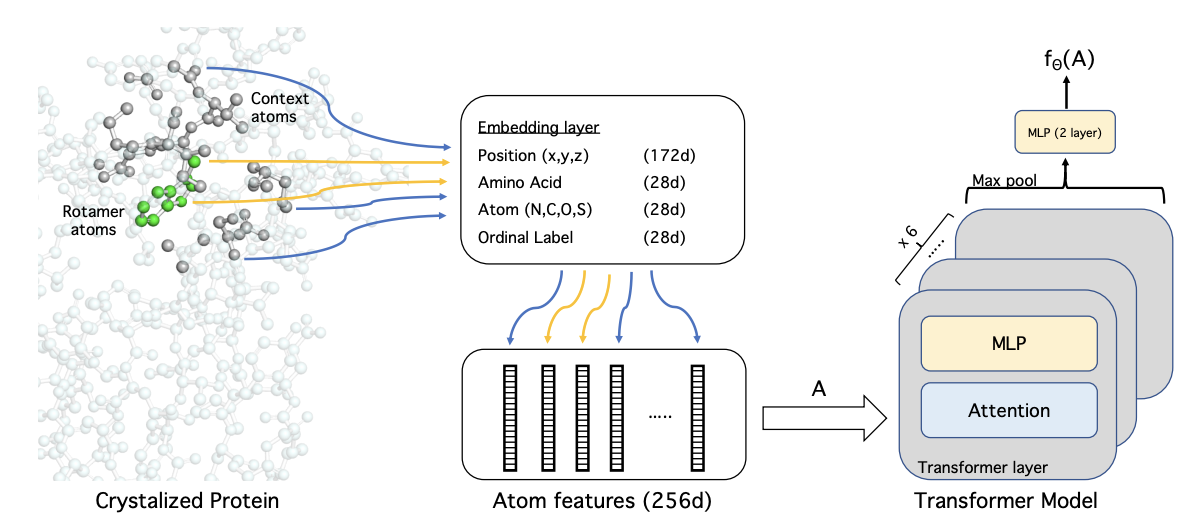We introduce EBMs for modeling the underlying energy landscape of atomic level protein conformations. We train EBMs to predict the energy of different protein rotamer configurations, and find that our trained EBM models can nearly match the performance of classical energy function Rosetta on the task of protein sidechain prediction.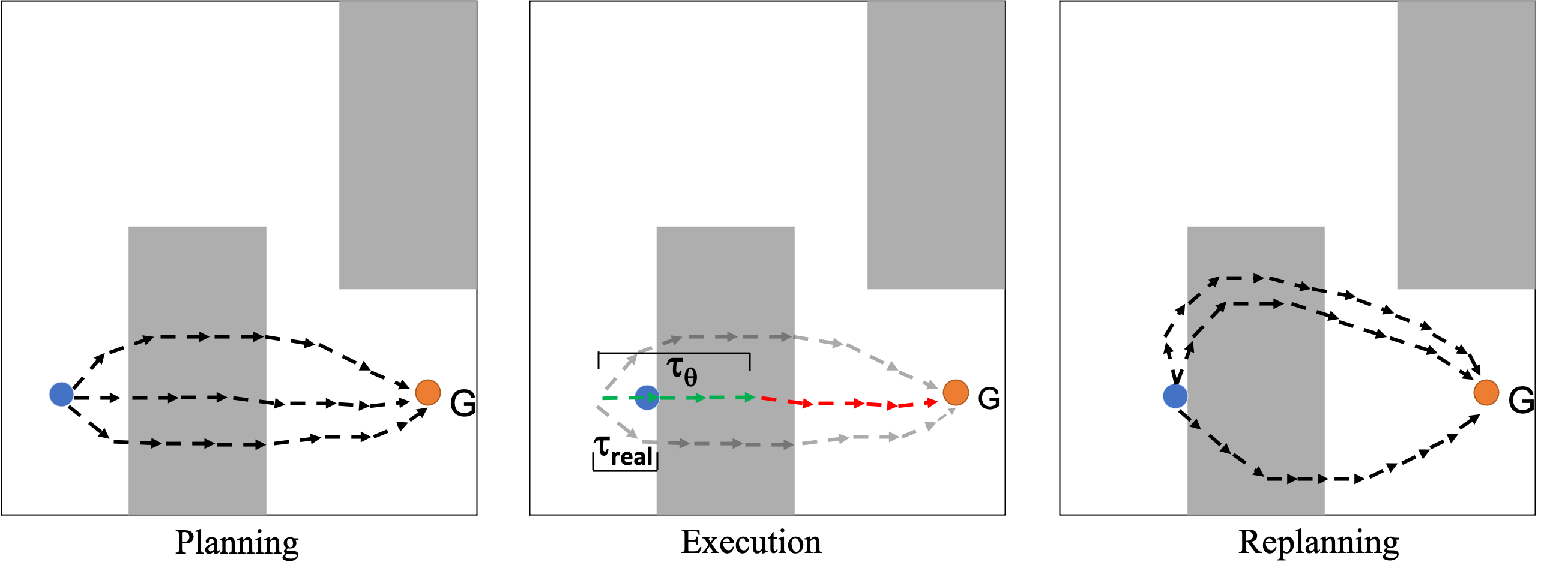We present a framework towards utilizing EBMs to learn, in an online fashion, trajectory level plans for different start and goal configurations. This allows us to flexibly change and adapt to different sets of goals by changing the underlying trajectory inference objective.
We introduce a method to scale EBM training to modern neural network architectures. We show that such trained EBMs have a set of unique properties, enabling model robustness, image and trajectory modeling, continual learning and compositional visual generation.

## Bibtex

@inproceedings{du2021improved, title={Improved Contrastive Divergence Training of Energy Based Models}, author={Du, Yilun and Li, Shuang and Tenenbaum, B. Joshua and Mordatch, Igor}, booktitle={Proceedings of the 38th International Conference on Machine Learning (ICML-21)}, year={2021} }

Send feedback and questions to Yilun Du